Next: Experiments Up: Variational Bayesian learning for Previous: Variational Bayesian learning for

## Nonlinear factor analysis and hierarchical nonlinear factor analysis

In , a nonlinear generative model (1) was estimated by ensemble learning and the method was called nonlinear factor analysis (NFA). A more recent version with an analytical cost function and a linear computational complexity, is called hierarchical nonlinear factor analysis (HNFA) . In many respects HNFA is similar to NFA. The posterior approximation, for instance, was chosen to be maximally factorial for the sake of computational efficiency and the termswere restricted to be Gaussian.

In NFA, a multi-layer perceptron (MLP) network with one hidden layer was used for modelling the nonlinear mapping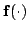:(3)

where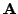and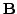are weight matrices,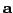andare bias vectors and the activation function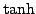operates on each element separately. The key idea in HNFA is to introduce latent variables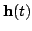before the nonlinearities and thus split the mapping (3) into two parts: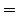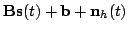(4)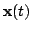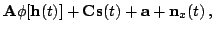(5)

where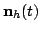andare Gaussian noise terms and the nonlinearityagain operates on each element separately. Note that we have included a short-cut mapping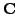from sources to observations. This means that hidden nodes only need to model the deviations from linearity.

Learning is unsupervised and thus differs in many ways from standard backpropagation. Each step in learning tries to minimise the cost function (2). In NFA, the sources are updated while keeping the mapping constant and vice versa. The computational complexity is proportional to the number of paths from sources to the data, i.e. the product of sizes of the three layers. In HNFA, all termsofare updated one at a time. The computational complexity is linear with the number of connections in the model and thus HNFA scales better than NFA. In both algorithms, the update steps are repeated for several thousands of times per parameter.

In NFA, neither the posterior mean nor the variance ofovercan be computed analytically. The approximation based on Taylor series expansion may be inaccurate if the posterior variance for the input of the hidden nodes grows too large. This may be the source of the instability observed in some simulations. Preliminary experiments suggest that it may be possible to fix the problem at the expense of efficiency.

In HNFA, the posterior mean and variance of the mappings in (4) and (5) have analytic expressions. This is possible at the expense of assuming independencies of the extra latent variablesin the posterior approximation. The assumption increases the misfit between the approximated and the true posterior. Minimisation of (2) pushes the solution in a direction where the misfit would be smaller. In , it is shown how this can lead to suboptimal separation in linear ICA. It is difficult to analyse the situation in nonlinear models, but it can be expected that models with fewer simultaneously active hidden nodes and thus more linear mappings are favoured. This should lead to conservative estimates of the nonlinearity of the model.

Since HNFA is built from simple blocks introduced in , learning the structure2 becomes easier. The cost function (2) relates to the model evidenceand can thus be used to compare structures. The model is built in stages starting from linear FA, i.e. HNFA without hidden nodes. See  for further details.Next: Experiments Up: Variational Bayesian learning for Previous: Variational Bayesian learning for
Tapani Raiko 2003-07-01